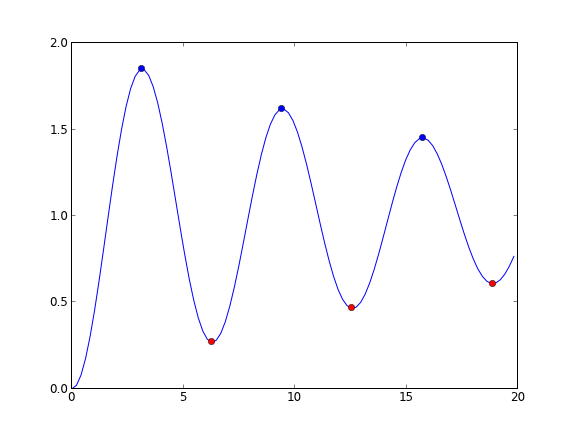## Finding minima and maxima in ODE solutions with events

| categories: ode | tags: | View Comments

Matlab post Today we look at another way to use events in an ode solver. We use an events function to find minima and maxima, by evaluating the ODE in the event function to find conditions where the first derivative is zero, and approached from the right direction. A maximum is when the fisrt derivative is zero and increasing, and a minimum is when the first derivative is zero and decreasing.

We use a simple ODE, $$y' = sin(x)*e^{-0.05x}$$, which has minima and maxima.

from pycse import *
import numpy as np

def ode(y, x):
return np.sin(x) * np.exp(-0.05 * x)

def minima(y, x):
'''Approaching a minumum, dydx is negatime and going to zero. our event function is increasing'''
value = ode(y, x)
direction = 1
isterminal = False
return value,  isterminal, direction

def maxima(y, x):
'''Approaching a maximum, dydx is positive and going to zero. our event function is decreasing'''
value = ode(y, x)
direction = -1
isterminal = False
return value,  isterminal, direction

xspan = np.linspace(0, 20, 100)

y0 = 0

X, Y, XE, YE, IE = odelay(ode, y0, xspan, events=[minima, maxima])
print IE
import matplotlib.pyplot as plt
plt.plot(X, Y)

# blue is maximum, red is minimum
colors = 'rb'
for xe, ye, ie in zip(XE, YE, IE):
plt.plot([xe], [ye], 'o', color=colors[ie])

plt.savefig('./images/ode-events-min-max.png')
plt.show()

[1, 0, 1, 0, 1, 0]Copyright (C) 2013 by John Kitchin. See the License for information about copying.

org-mode source